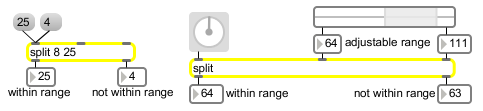split

Look for a range of numbers

Description

split looks to see if any input can fall within a specified range. If it does fall within that range, it sends the number out the left outlet and if it doesn't fall within the specified range, then it sends the number out the right outlet.

Arguments

Name Type Opt Description
minimum and maximum int or float Optional. The first argument sets the minimum value to be sent out the left outlet. If the first argument is an int, then the split object will convert all float values to ints. The second argument sets the maximum value to be sent out the left outlet. If the first argument to split is an int, the output is int. If it is float, the output is float. This is true regardless of the type of the input.

Messages

 int input [int] In left inlet: If the number is within a specified range, it is sent out the left outlet. Otherwise, it is sent out the right outlet. In middle inlet: The number is stored as the minimum value in the range of numbers looked for by split. If the number is an int, then the split object will convert all float values to ints. In right inlet: The number is stored as the maximum value in the range of numbers looked for by split. float input [float] In left inlet: If the number is within a specified range, it is sent out the left outlet. Otherwise, it is sent out the right outlet. In middle inlet: The number is stored as the minimum value in the range of numbers looked for by split. If the number is an int, then the split object will convert all float values to ints. In right inlet: The number is stored as the maximum value in the range of numbers looked for by split.

Output

int: If the number received in the left inlet is greater than or equal to the specified minimum, and it is less than or equal to the specified maximum, it is sent out the left outlet. Otherwise, it is sent out the right outlet.

ExamplesUsed to divert a certain range of numbers to a different destination